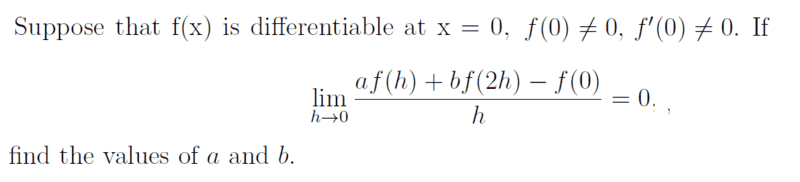# Find the values of a and b in a limit

• songoku

#### songoku

Homework Statement
Relevant Equations
Limit

L'Hopital Rule is not allowedI know $$\lim_{h\rightarrow 0} af(h)+bf(2h)−f(0)=0$$
$$a+b=1$$

But I don't know how to find the second equation involving a and b. I imagine I need to somehow obtain ##h## in numerator so I can cross out with ##h## in denominator but I don't have idea how to get ##h## in the numerator.

Thanks

Try using Taylor's theorem to express ##f(h)## as a linear polynomial in ##h## plus an error term that decreases superlinearly, and do the same for ##f(2h)##.

•songoku and topsquark
Try using Taylor's theorem to express ##f(h)## as a linear polynomial in ##h## plus an error term that decreases superlinearly, and do the same for ##f(2h)##.
Based on the definition of derivative that he gave in another thread, I think this is not the approach that is expected.

•songoku and topsquark
Based on the definition of derivative that he gave in another thread, I think this is not the approach that is expected.
Yes, my lesson has not covered Taylor's theorem

Try using Taylor's theorem to express ##f(h)## as a linear polynomial in ##h## plus an error term that decreases superlinearly, and do the same for ##f(2h)##.
I tried using Taylor's theorem, as suggested, as best as I can and by only taking the term up until ##h## I get ##a+2b=0##

Is there other possible method to solve the question without using Taylor?

Thanks

Yes, my lesson has not covered Taylor's theorem

I tried using Taylor's theorem, as suggested, as best as I can and by only taking the term up until ##h## I get ##a+2b=0##

Is there other possible method to solve the question without using Taylor?

Thanks
Why not do the obvious decomposition of the limit into derivatives of ##f##?

•SammyS and songoku
Why not do the obvious decomposition of the limit into derivatives of ##f##?
I think I understand this hint.

Thank you very much for the help Office_Shredder, FactChecker, PeroK

Why not do the obvious decomposition of the limit into derivatives of ##f##?

I think I understand this hint.
Just in case you don't quite understand that hint, or the advice from @FactChecker in Post #3 ...

You had the following as one of the definitions for ##f'(x_0)##.

##\displaystyle f'(x_0)=\lim_{h \to 0} \frac{f(x_0+h)-f(x_0)}{h} ##

So that ##\displaystyle \ f'(0)=\lim_{h \to 0} \frac{f(h)-f(0)}{h} \ .##

You may not realize that ##\displaystyle \ \lim_{h \to 0} \frac{f(2h)-f(0)}{2h} = f'(0)\ ## as well.

(Added a short time later with the Edit feature):

In decomposing the given limit, take the ##\displaystyle \frac{b\,f(2h)}{h} ## term, for instance and write it as ##\displaystyle \frac{b\,(f(2h)-f(0))}{h} + \frac{b\,(f(0))}{h} \ .##

Do similarly with ##\displaystyle \frac{a\,f(h)}{h}##, combine like terms, etc.

Last edited:
•songoku